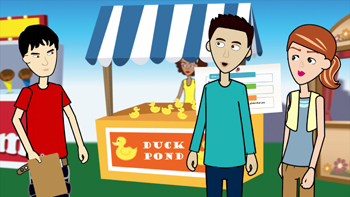# Welcome!In this module, you will have some fun looking at games and the probability of ‘“winning” prizes. By studying the probability of winning, you will try and increase your chances of winning.

In this module, you will:

• Find and interpret probabilities.
• Explore conditional probabilities, and use the conditional probability to determine if two events are independent.
• Work with two-way tables as you analyze conditional probabilities and possible outcomes.
• Use your knowledge of probability to maximize the probability of desired outcomes.
To find other Algebra II modules, go to Common Core Algebra II Statistics and Probability.

# Lessons

## Prize Winner, Part 1

In this lesson, you will find and interpret conditional probabilities. You will look at various simple carnival games, and determine the probabilities of winning. Winning the games involves conditional probabilities. You should have knowledge of simple probability, including finding the probability of independent and dependent events.

> Go to Lesson: Prize Winner, Part 1

## Prize Winner, Part 2

This lesson applies what you know about probability and conditional probability, using two-way tables.

> Go to Lesson: Prize Winner, Part 2

## Prize Winner, Part 3

This lesson is a summary lesson in which you will use your knowledge of probability to make informed decisions. You will use unions, intersections and complements to compute and interpret theoretical and experimental probabilities of compound events, attending to mutually exclusive events, independent events and conditional probabilities.

> Go to Lesson: Prize Winner, Part 3